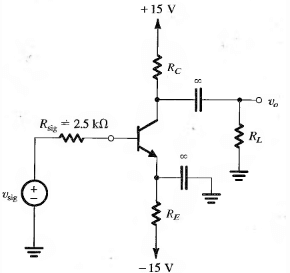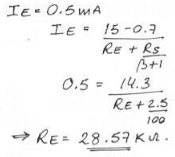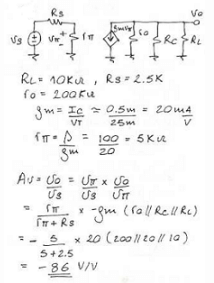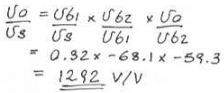# Test: Basic BJT Amplifier Configuration

## 10 Questions MCQ Test Analog Circuits | Test: Basic BJT Amplifier Configuration

Description
Attempt Test: Basic BJT Amplifier Configuration | 10 questions in 10 minutes | Mock test for Electronics and Communication Engineering (ECE) preparation | Free important questions MCQ to study Analog Circuits for Electronics and Communication Engineering (ECE) Exam | Download free PDF with solutions
QUESTION: 1

### (Q1-Q.6) An amplifier is measured to have an internal resistance of 10 kΩ, voltage gain of 100V/V and output resistance of 100 Ω. Also, when a load resistance of 1 kΩ is connected between the output resistance if found to decrease to 8 kΩ. If the amplifier is fed with the signal source having an internal resistance of 2 kΩ, then Q. Find Gm.

Solution:

Gm = (voltage gain in V/V) / (output resistance) or 100/100 A/V.

QUESTION: 2

### Find Av.

Solution:

Av = Avo X Rl/Ro+Rl or 100 X 1000/1000+100 or 90.9 V/V.

QUESTION: 3

### Find Gvo.

Solution:

Gvo = (Avo X input resistance) / (input resistance + signal resistance).

QUESTION: 4

Find Gv.

Solution:

Gv = (Gvo X Av) / Avo or 83.3 X 90.9 / 100 V/V.

QUESTION: 5

Find R out.

Solution:

Rout = Rl (1 – Gvo/Gv). Put in the respective values and solve.

QUESTION: 6

Find Ai.

Solution:

(Vo X R in) / (Vi X Rl) gives the required value of Ai.

QUESTION: 7

(Q.7-Q.10) The circuit shown below is a small sine wave signal with average zero and transistor ß =Q. Find the value of R(E) to establish a dc emitter current of about 0.5 mA.

Solution:QUESTION: 8

Find R(C) to establish a dc collector voltage of about +5V.

Solution:

Vc = 15 – Rc.Ic
5 = 15 – Rc * 0.99 * 0.5m
Rc = 20.2kΩ
= 20kΩ.

QUESTION: 9

For R (L) = 10 kΩ and transistor Ro = 200 kΩ, determine the overall voltage gain.

Solution:QUESTION: 10

Find the overall voltage gain.

Solution:Use Code STAYHOME200 and get INR 200 additional OFF Use Coupon Code